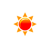# BTU to Kilowatt-hour Converter (btu to kWh)

 Example: 1 btu = 0.00029307107 kilowatt hours BTU: btu kWh: kWh
You may also interested in: Kilowatt-hour to BTU Converter

The online btu to kWh Converter is used to convert the energy from btu to kWh.

#### The BTU to Kilowatt-hour Conversion Formula

You can use the following formula to convert from miles to km :

X(kWh) = y(BTU) × 0.00029307107017

Example: How to convert 1000 btu to kilowatt-hour ?

X(kWh) = 1000(BTU) × 0.00029307107017

#### BTU to Kilowatt-hour conversion table


BTU (btu) Kilowatt-hour (kWh)
1 btu0.000293 kWh
2 btu0.000586 kWh
3 btu0.000879 kWh
4 btu0.001172 kWh
5 btu0.001465 kWh
6 btu0.001758 kWh
7 btu0.002051 kWh
8 btu0.002345 kWh
9 btu0.002638 kWh
10 btu0.002931 kWh
20 btu0.005861 kWh
30 btu0.008792 kWh
40 btu0.011723 kWh
50 btu0.014654 kWh
60 btu0.017584 kWh
70 btu0.020515 kWh
80 btu0.023446 kWh
90 btu0.026376 kWh
100 btu0.029307 kWh
500 btu0.146536 kWh
1000 btu0.293071 kWh

To know how to convert BTU to kilowatt hours, please use our BTU to kilowatt hours Converter for free.

#### References

More references for BTU and kilowatt hours

#### Local Weather Status

Ashburn, US
13th November, 2019 Wednesday
Clear Sky-5 - -1.67
Humidity: 63 %
Wind: 6.2 km/h